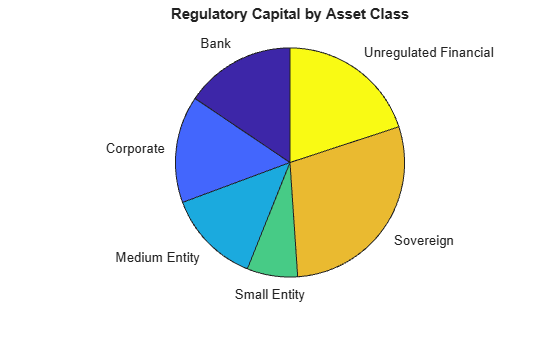Documentation

## Calculating Regulatory Capital with the ASRF Model

This example shows how to calculate capital requirements and value-at-risk (VaR) for a credit sensitive portfolio of exposures using the asymptotic single risk factor (ASRF) model. This example also shows how to compute Basel capital requirements using an ASRF model.

### The ASRF Model

The ASRF model defines capital as the credit value at risk (VaR) in excess of the expected loss (EL).

`$capital=VaR-EL$`

where the EL for a given counterparty is the exposure at default (EAD) multiplied by the probability of default (PD) and the loss given default (LGD).

`$EL=EAD*PD*LGD$`

To compute the credit VaR, the ASRF model assumes that obligor credit quality is modeled with a latent variable (A) using a one factor model where the single common factor (Z) represents systemic credit risk in the market.

`${A}_{i}=\sqrt{{\rho }_{i}}\cdot Z+\sqrt{1-{\rho }_{i}}\cdot ϵ$`

Under this model, default losses for a particular scenario are calculated as:

`$L=EAD\cdot I\cdot LGD$`

where I is the default indicator, and has a value of 1 if ${A}_{i}<{\Phi }_{A}^{-1}\left(P{D}_{i}\right)$ (meaning the latent variable has fallen below the threshold for default), and a value of 0 otherwise. The expected value of the default indicator conditional on the common factor is given by:

`$E\left({I}_{i}|Z\right)={\Phi }_{ϵ}\left(\frac{{\Phi }_{A}^{-1}\left(P{D}_{i}\right)-\sqrt{{\rho }_{i}}Z}{\sqrt{1-{\rho }_{i}}}\right)$`

For well diversified and perfectly granular portfolios, the expected loss conditional on a value of the common factor is:

`$L|Z=\sum _{i}EA{D}_{i}\cdot LG{D}_{i}\cdot {\Phi }_{ϵ}\left(\frac{{\Phi }_{A}^{-1}\left(P{D}_{i}\right)-\sqrt{{\rho }_{i}}Z}{\sqrt{1-{\rho }_{i}}}\right)$`

You can then directly compute particular percentiles of the distribution of losses using the cumulative distribution function of the common factor. This is the credit VaR, which we compute at the $\alpha$ confidence level:

`$creditVaR\left(\alpha \right)=\sum _{i}EA{D}_{i}\cdot LG{D}_{i}\cdot {\Phi }_{ϵ}\left(\frac{{\Phi }_{A}^{-1}\left(P{D}_{i}\right)-\sqrt{{\rho }_{i}}{\Phi }_{Z}^{-1}\left(1-\alpha \right)}{\sqrt{1-{\rho }_{i}}}\right)$`

It follows that the capital for a given level of confidence, $\alpha$, is:

`$capital\left(\alpha \right)=\sum _{i}EA{D}_{i}\cdot LG{D}_{i}\cdot \left[{\Phi }_{ϵ}\left(\frac{{\Phi }_{A}^{-1}\left(P{D}_{i}\right)-\sqrt{{\rho }_{i}}{\Phi }_{Z}^{-1}\left(1-\alpha \right)}{\sqrt{1-{\rho }_{i}}}\right)-P{D}_{i}\right]$`

### Basic ASRF

The portfolio contains 100 credit sensitive contracts and information about their exposure. This is simulated data.

```load asrfPortfolio.mat disp(portfolio(1:5,:))```
``` ID EAD PD LGD AssetClass Sales Maturity __ __________ _________ ____ __________ _____ ____________________ 1 2.945e+05 0.013644 0.5 "Bank" NaN 02-Jun-2023 00:00:00 2 1.3349e+05 0.0017519 0.5 "Bank" NaN 05-Jul-2021 00:00:00 3 3.1723e+05 0.01694 0.4 "Bank" NaN 07-Oct-2018 00:00:00 4 2.8719e+05 0.013624 0.35 "Bank" NaN 27-Apr-2022 00:00:00 5 2.9965e+05 0.013191 0.45 "Bank" NaN 07-Dec-2022 00:00:00 ```

The asset correlations ($\rho$) in the ASRF model define the correlation between similar assets. The square root of this value, $\sqrt{\rho }$, specifies the correlation between a counterparty's latent variable (A) and the systemic credit factor (Z). Asset correlations can be calibrated by observing correlations in the market or from historical default data. Correlations can also be set using regulatory guidelines (see Basel Capital Requirements section).

Because the ASRF model is a fast, analytical formula, it is convenient to perform sensitivity analysis for a counterparty by varying the exposure parameters and observing how the capital and VaR change.

The following plot shows the sensitivity to PD and asset correlation. The LGD and EAD parameters are scaling factors in the ASRF formula so the sensitivity is straightforward.

```% Counterparty ID id = 1; % Set default asset correlation to 0.2 as baseline R = 0.2; % Compute baseline capital and VaR [capital0, var0] = asrf(portfolio.PD(id),portfolio.LGD(id),R,'EAD',portfolio.EAD(id)); % Stressed PD by 50% [capital1, var1] = asrf(portfolio.PD(id) * 1.5,portfolio.LGD(id),R,'EAD',portfolio.EAD(id)); % Stressed Correlation by 50% [capital2, var2] = asrf(portfolio.PD(id),portfolio.LGD(id),R * 1.5,'EAD',portfolio.EAD(id)); c = categorical({'ASRF Capital','VaR'}); bar(c,[capital0 capital1 capital2; var0 var1 var2]); legend({'baseline','stressed PD','stressed R'},'Location','northwest') title(sprintf('ID: %d, Baseline vs. Stressed Scenarios',id)); ylabel('USD (\$)');```### Basel Capital Requirements

When computing regulatory capital, the Basel documents have additional model specifications on top of the basic ASRF model. In particular, Basel II/III defines specific formulas for computing the asset correlation for exposures in various asset classes as a function of the default probability.

To set up the vector of correlations according to the definitions established in Basel II/III:

```R = zeros(height(portfolio),1); % Compute correlations for corporate, sovereign, and bank exposures idx = portfolio.AssetClass == "Corporate" |... portfolio.AssetClass == "Sovereign" |... portfolio.AssetClass == "Bank"; R(idx) = 0.12 * (1-exp(-50*portfolio.PD(idx))) / (1-exp(-50)) +... 0.24 * (1 - (1-exp(-50*portfolio.PD(idx))) / (1-exp(-50))); % Compute correlations for small and medium entities idx = portfolio.AssetClass == "Small Entity" |... portfolio.AssetClass == "Medium Entity"; R(idx) = 0.12 * (1-exp(-50*portfolio.PD(idx))) / (1-exp(-50)) +... 0.24 * (1 - (1-exp(-50*portfolio.PD(idx))) / (1-exp(-50))) -... 0.04 * (1 - (portfolio.Sales(idx)/1e6 - 5) / 45); % Compute correlations for unregulated financial institutions idx = portfolio.AssetClass == "Unregulated Financial"; R(idx) = 1.25 * 0.12 * (1-exp(-50*portfolio.PD(idx))) / (1-exp(-50)) +... 0.24 * (1 - (1-exp(-50*portfolio.PD(idx))) / (1-exp(-50)));```

Find the basic ASRF capital using the Basel-defined asset correlations. The default value for the VaR level is 99.9%.

`asrfCapital = asrf(portfolio.PD,portfolio.LGD,R,'EAD',portfolio.EAD);`

Additionally, the Basel documents specify a maturity adjustment to be added to each capital calculation. Here we compute the maturity adjustment and update the capital requirements.

```maturityYears = years(portfolio.Maturity - settle); b = (0.11852 - 0.05478 * log(portfolio.PD)).^2; maturityAdj = (1 + (maturityYears - 2.5) .* b) ./ (1 - 1.5 .* b); regulatoryCapital = asrfCapital .* maturityAdj; fprintf('Portfolio Regulatory Capital : \$%.2f\n',sum(regulatoryCapital));```
```Portfolio Regulatory Capital : \$2310819.05 ```

Risk weighted assets (RWA) are calculated as capital * 12.5.

```RWA = regulatoryCapital * 12.5; results = table(portfolio.ID,portfolio.AssetClass,RWA,regulatoryCapital,'VariableNames',... {'ID','AssetClass','RWA','Capital'}); % Results table disp(results(1:5,:))```
``` ID AssetClass RWA Capital __ __________ __________ _______ 1 "Bank" 4.7766e+05 38213 2 "Bank" 79985 6398.8 3 "Bank" 2.6313e+05 21050 4 "Bank" 2.9449e+05 23560 5 "Bank" 4.1544e+05 33235 ```

Aggregate the regulatory capital by asset class.

```assetClasses = unique(results.AssetClass); assetClassCapital = zeros(numel(assetClasses),1); for i = 1:numel(assetClasses) assetClassCapital(i) = sum(results.Capital(results.AssetClass == assetClasses(i))); end pie(assetClassCapital,cellstr(assetClasses)) title('Regulatory Capital by Asset Class');``````capitalTable = table(assetClasses, assetClassCapital,'VariableNames',{'AssetClass','Capital'}); disp(capitalTable);```
``` AssetClass Capital _______________________ __________ "Bank" 3.6894e+05 "Corporate" 3.5811e+05 "Medium Entity" 3.1466e+05 "Small Entity" 1.693e+05 "Sovereign" 6.8711e+05 "Unregulated Financial" 4.127e+05 ```